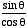index: click on a letter A B C D E F G H I J K L M N O P Q R S T U V W X Y Z A to Z index index: subject areas numbers & symbols sets, logic, proofs geometry algebra trigonometry advanced algebra & pre-calculus calculus advanced topics probability & statistics real world applications multimedia entrieswww.mathwords.com about mathwords website feedback

 tangent tan The trig function tangent, written tan θ. tan θ equals. For acute angles, tan θ can be found by the SOHCAHTOA definition as shown below on the left. The circle definition, a generalization of SOHCAHTOA, is shown below on the right. f(x) = tan x is a periodic function with period π.See also